## Three Address Code | DAGs | Basic Blocks & Flow Graphs-

Before you go through this article, make sure that you have gone through the previous articles on-

In this article, we will solve Miscellaneous Problems based on these Concepts.

## Problem-01:

Generate three address code for the following code-

c = 0

do

{

if (a < b) then

x++;

else

x–;

c++;

} while (c < 5)

## Solution-

Three address code for the given code is-

1. c = 0
2. if (a < b) goto (4)
3. goto (7)
4. T1 = x + 1
5. x = T1
6. goto (9)
7. T2 = x – 1
8. x = T2
9. T3 = c + 1
10. c = T3
11. if (c < 5) goto (2)

## Problem-02:

Generate three address code for the following code-

while (A < C and B > D) do

if A = 1 then C = C + 1

else

while A <= D

do A = A + B

## Solution-

Three address code for the given code is-

1. if (A < C) goto (3)
2. goto (15)
3. if (B > D) goto (5)
4. goto (15)
5. if (A = 1) goto (7)
6. goto (10)
7. T1 = c + 1
8. c = T1
9. goto (1)
10. if (A <= D) goto (12)
11. goto (1)
12. T2 = A + B
13. A = T2
14. goto (10)

## Problem-03:

Generate three address code for the following code-

switch (ch)

{

case 1 : c = a + b;

break;

case 2 : c = a – b;

break;

}

## Solution-

Three address code for the given code is-

if ch = 1 goto L1

if ch = 2 goto L2

L1:

T1 = a + b

c = T1

goto Last

L2:

T1 = a – b

c = T2

goto Last

Last:

## Problem-04:

Construct a DAG for the following three address code-

1. a = b + c
2. t1 = a x a
3. b = t1 + a
4. c = t1 x b
5. t2 = c + b
6. a = t2 + t2

## Solution-

Directed acyclic graph for the given three address code is-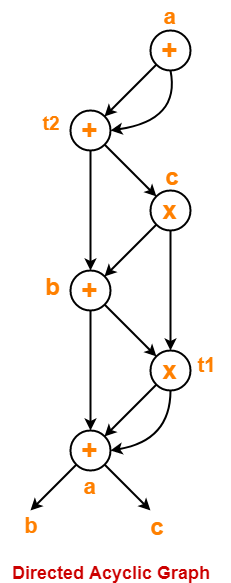## Problem-05:

Consider the following code-

prod = 0 ;

i = 1 ;

do

{

prod = prod + a[ i ] x b[ i ] ;

i = i + 1 ;

} while (i <= 10) ;

1. Compute the three address code.
2. Compute the basic blocks and draw the flow graph.

## Solution-

### Part-01:

Three address code for the given code is-

prod = 0

i = 1

T1 = 4 x i

T2 = a[T1]

T3 = 4 x i

T4 = b[T3]

T5 = T2 x T4

T6 = T5 + prod

prod = T6

T7 = i + 1

i = T7

if (i <= 10) goto (3)

### Step-01:

We identify the leader statements as-

• prod = 0 is a leader because first statement is a leader.
• T1 = 4 x i is a leader because target of conditional or unconditional goto is a leader.

### Step-02:

The above generated three address code can be partitioned into 2 basic blocks as-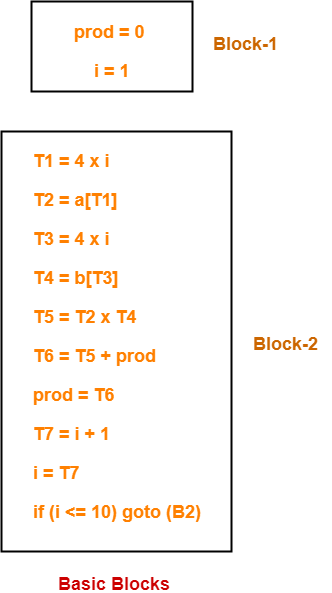### Step-03:

The flow graph is-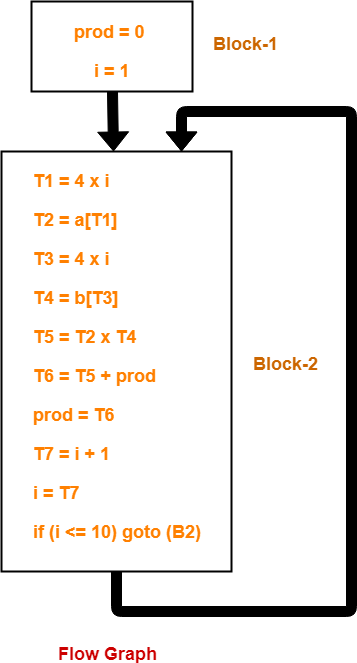To gain better understanding about these Miscellaneous Problems,

Watch this Video LectureNext Article- Code Optimization

Get more notes and other study material of Compiler Design.

Watch video lectures by visiting our YouTube channel LearnVidFun.

## Quadruples, Triples and Indirect Triples

Before you go through this article, make sure that you have gone through the previous article on Three Address Code.

We have discussed-

• Three Address Code is a form of an intermediate code.
• It is generated by the compiler for implementing Code Optimization.
• It uses maximum three addresses to represent any statement.

## Implementation-

 Three Address Code is implemented as a record with the address fields.

The commonly used representations for implementing Three Address Code are-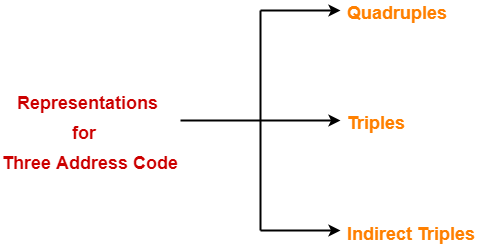2. Triples
3. Indirect Triples

In quadruples representation, each instruction is splitted into the following 4 different fields-

op, arg1, arg2, result

Here-

• The op field is used for storing the internal code of the operator.
• The arg1 and arg2 fields are used for storing the two operands used.
• The result field is used for storing the result of the expression.

## Exceptions

There are following exceptions-

### Exception-01:

To represent the statement x = op y, we place-

• op in the operator field
• y in the arg1 field
• x in the result field
• arg2 field remains unused

### Exception-02:

To represent the statement like param t1, we place-

• param in the operator field
• t1 in the arg1 field
• Neither arg2 field nor result field is used

### Exception-03:

To represent the unconditional and conditional jump statements, we place label of the target in the result field.

## 2. Triples-

In triples representation,

• References to the instructions are made.
• Temporary variables are not used.

## 3. Indirect Triples-

• This representation is an enhancement over triples representation.
• It uses an additional instruction array to list the pointers to the triples in the desired order.
• Thus, instead of position, pointers are used to store the results.
• It allows the optimizers to easily re-position the sub-expression for producing the optimized code.

## Problem-01:

Translate the following expression to quadruple, triple and indirect triple-

a + b x c / e ↑ f + b x c

## Solution-

Three Address Code for the given expression is-

T1 = e ↑ f

T2 = b x c

T3 = T2 / T1

T4 = b x a

T5 = a + T3

T6 = T5 + T4

Now, we write the required representations-

 Location Op Arg1 Arg2 Result (0) ↑ e f T1 (1) x b c T2 (2) / T2 T1 T3 (3) x b a T4 (4) + a T3 T5 (5) + T5 T4 T6

### Triple Representation-

 Location Op Arg1 Arg2 (0) ↑ e f (1) x b c (2) / (1) (0) (3) x b a (4) + a (2) (5) + (4) (3)

### Indirect Triple Representation-

 Statement 35 (0) 36 (1) 37 (2) 38 (3) 39 (4) 40 (5)

 Location Op Arg1 Arg2 (0) ↑ e f (1) x b e (2) / (1) (0) (3) x b a (4) + a (2) (5) + (4) (3)

## Problem-02:

Translate the following expression to quadruple, triple and indirect triple-

a = b x – c + b x – c

## Solution-

Three Address Code for the given expression is-

T1 = uminus c

T2 = b x T1

T3 = uminus c

T4 = b x T3

T5 = T2 + T4

a = T5

Now, we write the required representations-

 Location Op Arg1 Arg2 Result (1) uminus c T1 (2) x b T1 T2 (3) uminus c T3 (4) x b T3 T4 (5) + T2 T4 T5 (6) = T5 a

### Triple Representation-

 Location Op Arg1 Arg2 (1) uminus c (2) x b (1) (3) uminus c (4) x b (3) (5) + (2) (4) (6) = a (5)

### Indirect Triple Representation-

 Statement 35 (1) 36 (2) 37 (3) 38 (4) 39 (5) 40 (6)

 Location Op Arg1 Arg2 (1) uminus c (2) x b (1) (3) uminus c (4) x b (3) (5) + (2) (4) (6) = a (5)

Watch this Video LectureNext Article- Basic Blocks and Flow Graphs

Get more notes and other study material of Compiler Design.

Watch video lectures by visiting our YouTube channel LearnVidFun.

## Three Address Code | Examples

 Three Address Code is a form of an intermediate code.

The characteristics of Three Address instructions are-

• They are generated by the compiler for implementing Code Optimization.
• They use maximum three addresses to represent any statement.
• They are implemented as a record with the address fields.

## General Form-

In general, Three Address instructions are represented as-

 a = b op c

Here,

• a, b and c are the operands.
• Operands may be constants, names, or compiler generated temporaries.
• op represents the operator.

### Examples-

Examples of Three Address instructions are-

• a = b + c
• c = a x b

## Common Three Address Instruction Forms-

The common forms of Three Address instructions are-

### 1. Assignment Statement-

 x = y op z and x = op y

Here,

• x, y and z are the operands.
• op represents the operator.

It assigns the result obtained after solving the right side expression of the assignment operator to the left side operand.

### 2. Copy Statement-

 x = y

Here,

• x and y are the operands.
• = is an assignment operator.

It copies and assigns the value of operand y to operand x.

### 3. Conditional Jump-

 If x relop y goto X

Here,

• x & y are the operands.
• X is the tag or label of the target statement.
• relop is a relational operator.

If the condition “x relop y” gets satisfied, then-

• The control is sent directly to the location specified by label X.
• All the statements in between are skipped.

If the condition “x relop y” fails, then-

• The control is not sent to the location specified by label X.
• The next statement appearing in the usual sequence is executed.

### 4. Unconditional Jump-

 goto X

Here, X is the tag or label of the target statement.

On executing the statement,

• The control is sent directly to the location specified by label X.
• All the statements in between are skipped.

### 5. Procedure Call-

 param x call p return y

Here, p is a function which takes x as a parameter and returns y.

## PRACTICE PROBLEMS BASED ON THREE ADDRESS CODE-

To solve the problems, Learn about the Precedence Relations and Associativity of Operators.

## Problem-01:

Write Three Address Code for the following expression-

a = b + c + d

## Solution-

The given expression will be solved as-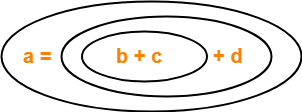Three Address Code for the given expression is-

(1) T1 = b + c

(2) T2 = T1 + d

(3) a = T2

## Problem-02:

Write Three Address Code for the following expression-

-(a x b) + (c + d) – (a + b + c + d)

## Solution-

Three Address Code for the given expression is-

(1) T1 = a x b

(2) T2 = uminus T1

(3) T3 = c + d

(4) T4 = T2 + T3

(5) T5 = a + b

(6) T6 = T3 + T5

(7) T7 = T4 – T6

## Problem-03:

Write Three Address Code for the following expression-

If A < B then 1 else 0

## Solution-

Three Address Code for the given expression is-

(1) If (A < B) goto (4)

(2) T1 = 0

(3) goto (5)

(4) T1 = 1

(5)

## Problem-04:

Write Three Address Code for the following expression-

If A < B and C < D then t = 1 else t = 0

### Solution-

Three Address Code for the given expression is-

(1) If (A < B) goto (3)

(2) goto (4)

(3) If (C < D) goto (6)

(4) t = 0

(5) goto (7)

(6) t = 1

(7)

Watch this Video LectureNext Article- Quadruples, Triples & Indirect Triples

Get more notes and other study material of Compiler Design.

Watch video lectures by visiting our YouTube channel LearnVidFun.